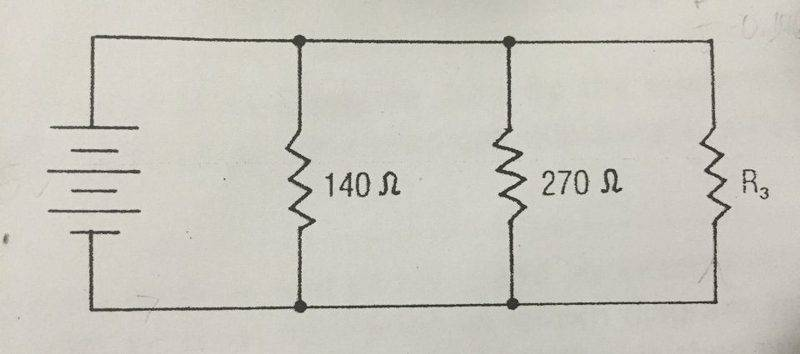# Finding a Missing Resistor

## Homework Statement

Three resistors are connected as shown in the diagram. If the current from the power supply is 250 mA and the current through R3 is 54 mA, what is the resistance of R3?## Homework Equations

1/Req= 1/R1 + 1/R2 +1/R3

V=IR

## The Attempt at a Solution

First I used the parallel circuit formula of 1/Req= 1/R1+ 1/R2 + 1/R3
but there are two variables (Req and R3) so I'm not sure how to use that. Also, there's no voltage provided so I'm a bit confused as how to go from here. Any help would be appreciated :)

cnh1995
Homework Helper
Gold Member
You can see that the resistors are in parallel, meaning voltage across each resistor is equal to the battery voltage.
current from the power supply is 250 mA
and the current through R3 is 54 mA,
Could you calculate voltage of the battery from this information?
Hint: Ohm's law and resistances in parallel.

Last edited:
•Aleena753
I had an idea for finding the solution. I was thinking that I could find the total V and then divide it by the total current to find Req and then use that Req in the parallel circuit formula (1/Req= 1/R1 + 1/R2 + 1/R3) to find R3. As for the total voltage of the battery, I know to use V=IR but I'm not sure which numbers to use, since the current of the two known resistors is unknown.

cnh1995
Homework Helper
Gold Member
since the current of the two known resistors is unknown.
Well, you can combine the two known resistances by finding their equivalent resistance. You can then calculate current through the equivalent resistance and apply Ohm's law.

So the current for the equivalent resistance of R1 and R2 would be the total current of the circuit with the current of the third resistor subtracted right?

cnh1995
Homework Helper
Gold Member
So the current for the equivalent resistance of R1 and R2 would be the total current of the circuit with the current of the third resistor subtracted right?
Right.

I had an idea for finding the solution. I was thinking that I could find the total V and then divide it by the total current to find Req and then use that Req in the parallel circuit formula (1/Req= 1/R1 + 1/R2 + 1/R3) to find R3. As for the total voltage of the battery, I know to use V=IR but I'm not sure which numbers to use, since the current of the two known resistors is unknown.[/QUOTE]
You can see that the resistors are in parallel, meaning voltage across each resistor is equal to the battery voltage.

Could you calculate voltage of the battery from this information?
Hint: Ohm's law and resistances in parallel.
Right.
So I found the voltage and then divided it by the third current (54mA) to find the resistance of the third resistor. I currently have what I believe is the right answer of 333 ohms.

cnh1995
Homework Helper
Gold Member
I currently have what I believe is the right answer of 333 ohms.
I'm getting 334.something ohms. But your method is correct.

I'm getting 334.something ohms. But your method is correct.
Alright thank you for your help : )